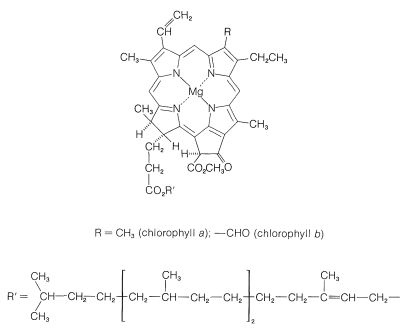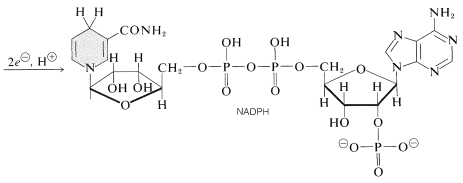# 20.10: Formation of Carbohydrates by Photosynthesis

$$\newcommand{\vecs}{\overset { \rightharpoonup} {\mathbf{#1}} }$$ $$\newcommand{\vecd}{\overset{-\!-\!\rightharpoonup}{\vphantom{a}\smash {#1}}}$$$$\newcommand{\id}{\mathrm{id}}$$ $$\newcommand{\Span}{\mathrm{span}}$$ $$\newcommand{\kernel}{\mathrm{null}\,}$$ $$\newcommand{\range}{\mathrm{range}\,}$$ $$\newcommand{\RealPart}{\mathrm{Re}}$$ $$\newcommand{\ImaginaryPart}{\mathrm{Im}}$$ $$\newcommand{\Argument}{\mathrm{Arg}}$$ $$\newcommand{\norm}{\| #1 \|}$$ $$\newcommand{\inner}{\langle #1, #2 \rangle}$$ $$\newcommand{\Span}{\mathrm{span}}$$ $$\newcommand{\id}{\mathrm{id}}$$ $$\newcommand{\Span}{\mathrm{span}}$$ $$\newcommand{\kernel}{\mathrm{null}\,}$$ $$\newcommand{\range}{\mathrm{range}\,}$$ $$\newcommand{\RealPart}{\mathrm{Re}}$$ $$\newcommand{\ImaginaryPart}{\mathrm{Im}}$$ $$\newcommand{\Argument}{\mathrm{Arg}}$$ $$\newcommand{\norm}{\| #1 \|}$$ $$\newcommand{\inner}{\langle #1, #2 \rangle}$$ $$\newcommand{\Span}{\mathrm{span}}$$$$\newcommand{\AA}{\unicode[.8,0]{x212B}}$$

Carbohydrates are formed in green plants by photosynthesis, which is the chemical combination, or "fixation", of carbon dioxide and water by utilization of energy from the absorption of visible light. The overall result is the reduction of carbon dioxide to carbohydrate and the formation of oxygen:If the carbohydrate formed is cellulose, then the reaction in effect is the reverse of the burning of wood, and obviously requires considerable energy input.

Because of its vital character to life as we know it, photosynthesis has been investigated intensively and the general features of the process are now rather well understood. The principal deficiencies in our knowledge include just how the light absorbed by the plants is converted to chemical energy and the details of how the many complex enzyme-induced reactions involved take place.

The ingredients in green plants that carry on the work of photosynthesis are contained in highly organized, membrane-covered units called chloroplasts. The specific substances that absorb the light are the plant pigments, chlorophyll a and chlorophyll b, whose structures are shown in Figure 20-6. These highly conjugated substances are very efficient light absorbers, and the energy so gained is used in two separate processes, which are represented diagrammatically in Figure 20-7.Figure 20-6: The structure of chlorophyll a and chlorophyll b, showing cis-trans relationships of the substituents.Figure 20-7: Simplified representation of the photoreactions in photosynthesis. The oxidation of water is linked to the reduction of $$\ce{NADP}^\oplus$$ by an electron-transport chain (dashed line) that is coupled to ATP formation (photophosphorylation).

One photoprocess reduces nicotinamide adenine dinucleotide phosphate $$\left( \ce{NADP}^\oplus \right)$$ to $$\ce{NADPH}$$. These dinucleotides, shown below, differ from $$\ce{NAD}^\oplus$$ and $$\ce{NADH}$$ (Section 15-6C) in having a phosphate group at $$\ce{C_2}$$ of one of the ribose units. The oxidized form, $$\ce{NADP}^\oplus$$, behaves like $$\ce{NAD}^\oplus$$ and receives the equivalent of $$\ce{H}^\ominus$$ at $$\ce{C_4}$$ of the nicotinamide ring to form $$\ce{NADPH}$$:The other important photoreaction is oxidation of water to oxygen by the reaction:

$\ce{H_2O} \rightarrow 2 \ce{H}^\oplus + \frac{1}{2} \ce{O_2} + 2 \ce{e}^\ominus$

The oxygen formed clearly comes from $$\ce{H_2O}$$ and not from $$\ce{CO_2}$$, because photosynthesis in the presence of water labeled with $$\ce{^{18}O}$$ produces oxygen labeled with $$\ce{^{18}O}$$, whereas carbon dioxide labeled with $$\ce{^{18}O}$$ does not give oxygen labeled with $$\ce{^{18}O}$$. Notice that the oxidation of the water produces two electrons, and that the formation of $$\ce{NADPH}$$ from $$\ce{NADP}^\oplus$$ requires two electrons. These reactions occur at different locations within the chloroplasts and in the process of transferring electrons from the water oxidation site to the $$\ce{NADP}^\oplus$$ reduction site, adenosine diphosphate (ADP) is converted to adenosine triphosphate (ATP; see Section 15-5F for discussion between the importance of such phosphorylations). Thus electron transport between the two photoprocesses is coupled to phosphorylation. This process is called photophosphorylation (Figure 20-7).

The end result of the photochemical part of photosynthesis is the formation of $$\ce{O_2}$$, $$\ce{NADPH}$$, and ATP. Much of the oxygen is released to the atmosphere, but the $$\ce{NADPH}$$ and ATP are utilized in a series of dark reactions that achieve the reduction of carbon dioxide to the level of a a carbohydrate (fructose). A balanced equation is

$6 \ce{CO_2} + 12 \ce{NADPH} + 12 \ce{H}^\oplus \rightarrow \ce{C_6H_{12}O_6} + 12 \ce{NADP}^\oplus + 6 \ce{H_2O}$

The cycle of reactions that converts carbon dioxide to carbohydrates is called the Calvin cycle, after M. Calvin, who received the Nobel Prize in chemistry in 1961 for his work on determining the path of carbon in photosynthesis.

Carbon enters the cycle as carbon dioxide. The key reaction by which the $$\ce{CO_2}$$ is "fixed" involves enzymatic carboxylation of a pentose, $$D$$-ribulose 1,5-phosphate.$$^8$$A subsequent hydrolytic cleavage of the $$\ce{C_2}$$-$$\ce{C_3}$$ bond of the carboxylation product (this amounts to a reverse Claisen condensation; Section 18-8B) yields two molecules of $$D$$-3-phosphoglycerate.$$^9$$

In subsequent steps, ATP is utilized to phosphorylate the carboxyl group of 3-phosphoglycerate to create 1,3-diphosphoglycerate (a mixed anhydride of glyceric and phosphoric acids). This substance then is reduced by $$\ce{NADPH}$$ to glyceraldehyde 3-phosphate:Two glyceraldehyde 3-phosphates are utilized to build the six-carbon chain of fructose by an aldol condensation $$\left( \ce{C_3} + \ce{C_3} \rightarrow \ce{C_6} \right)$$, but the donor nucleophile in this reaction is the phosphate ester of dihydroxypropanone, which is an isomer of glyceraldehyde 3-phosphate. Rearrangement of the $$\ce{C_3}$$ aldose to the $$\ce{C_3}$$ ketose (of the type described in Section 20-2D) therefore precedes the aldol addition. (For a discussion of the mechanism of the enzymatic aldol reaction, see Section 17-3F.) The fructose 1,6-diphosphate formed is then hydrolyzed to fructose 6-phosphate:From what we have described thus far, only one atom of carbon has been added from the atmosphere, and although we have reached fructose, five previously reduced carbons were consumed in the process. Thus the plant has to get back a five-carbon sugar from a six-carbon sugar to perpetuate the cycle. Rather than split off one carbon and use that as a building block to construct other sugars, an amazing series of transformations is carried on that can be summarized by the following equations:These reactions have several features in common. They all involve phosphate esters of aldoses or ketoses, and they resemble aldol or reverse-aldol condensations. Their mechanisms will no be considered here, but are discussed more explicitly in Sections 20-10A, 20-10B, and 25-10. Their summation is $$\ce{C_6} + 3 \ce{C_3} \rightarrow 3 \ce{C_5}$$, which means that fructose 6-phosphate as the $$\ce{C_6}$$ component reacts with a total of three $$\ce{C_3}$$ units (two glyceraldehyde 3-phosphates and one dihydroxypropanone phosphate) to give, ultimately, three ribulose 5-phosphates. Although the sequence may seem complex, it avoids building up pentose or hexose chains one carbon at a time from one-carbon intermediates.

The Calvin cycle is completed by the phosphorylation of $$D$$-ribulose 5-phosphate with ATP. The resulting $$D$$-ribulose 1,5-diphosphate then is used to start the cycle again by combining with carbon dioxide. There is one sixth more fructose is used to build other carbohydrates, notably glucose, starch, and cellulose.

$$^8$$All of the reactions we will be discussing are mediated by enzymes, and we will omit henceforth explicit mention of this fact. But it should not be forgotten that these are all enzyme-induced processes, for which we have few, if any, laboratory reagents to duplicate on the particular compounds involved.

$$^9$$We will henceforth, in equations, designate the various acids we encounter as the phosphate and the carboxylate anions, although this is hardly reasonable at the pH values normal in living cells. Glyceric and phosphoric acids are only partially ionized at pH 7-8. However, it would be equally unrealistic to represent the acids as being wholly undissociated.